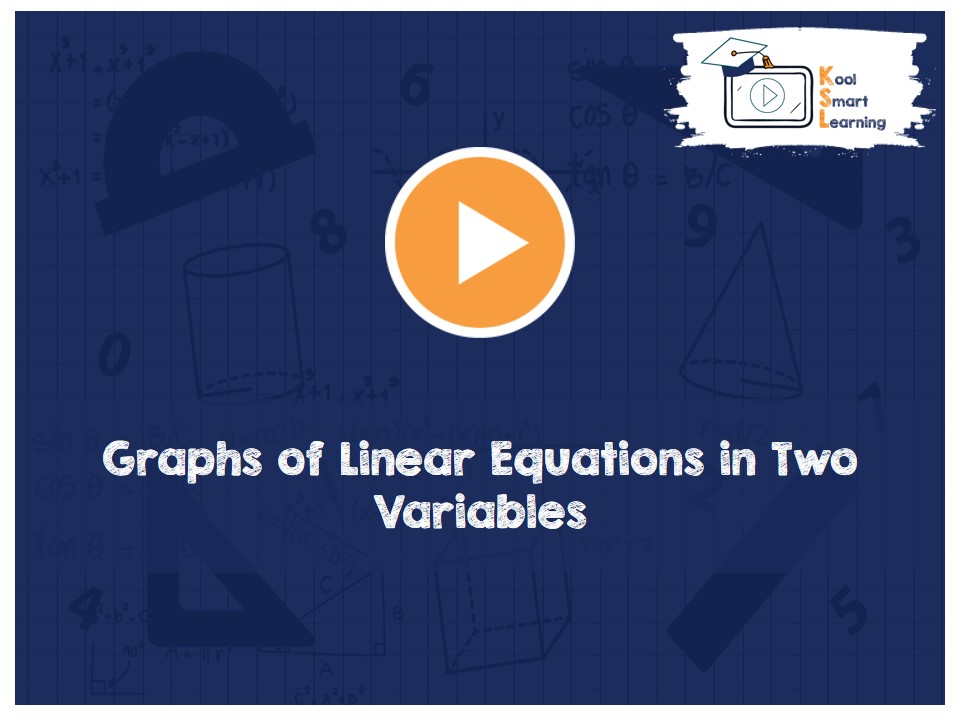At KoolSmartLearning, we intend to harness the power of online education to make learning easy.## Graphs of Linear Equations in Two Variables

/  Graphs of Linear Equations in Two Variables## Graphs of Linear Equations in Two Variables

This video talks about graphical representation of Linear Equations In Two Variables on the co-ordinate plane and finding the area between such graph and the co-ordinate axes. The video also describes what is the use of graphs of Linear Equations in Two Variables.

More Related Videos